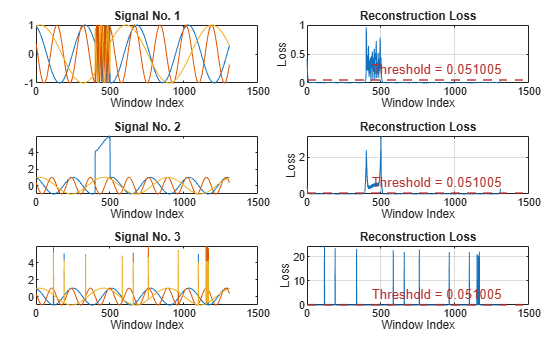# plotLoss

Plot window reconstruction loss

Since R2023a

## Syntax

``plotLoss(d,data)``
``plotLoss(d,data,Name=Value)``
``f = plotLoss(___)``

## Description

example

````plotLoss(d,data)` plots the window reconstruction loss computed over the input signal data set `data` by the detector `d`.```
````plotLoss(d,data,Name=Value)` specifies additional options using name-value arguments.```
````f = plotLoss(___)` returns the figure handle of the plot.```

## Examples

collapse all

Load a convolutional anomaly detector trained with three-channel sinusoidal signals. Display the model, threshold, and window properties of the detector.

```load sineWaveAnomalyDetector D```
```D = deepSignalAnomalyDetectorCNN with properties: IsTrained: 1 NumChannels: 3 Model Information ModelType: 'conv' FilterSize: 8 NumFilters: 32 NumDownsampleLayers: 2 DownsampleFactor: 2 DropoutProbability: 0.2000 Threshold Information Threshold: 0.0510 ThresholdMethod: 'contaminationFraction' ThresholdParameter: 0.0100 Window Information WindowLength: 1 OverlapLength: 'auto' WindowLossAggregation: 'mean' ```

Load the file `sineWaveAnomalyData.mat`, which contains two sets of synthetic three-channel sinusoidal signals.

• `sineWaveNormal` contains the 10 sinusoids used to train the convolutional anomaly detector. Each signal has a series of small-amplitude impact-like imperfections but otherwise has stable amplitude and frequency.

• `sineWaveAbnormal` contains three signals of similar length and amplitude to the training data. One of the signals has an abrupt, finite-time change in frequency. Another signal has a finite-duration amplitude change in one of its channels. A third has random spikes in each channel.

Plot three normal signals and the three signals with anomalies.

```load sineWaveAnomalyData tiledlayout(3,2,TileSpacing="compact",Padding="compact") rnd = randperm(length(sineWaveNormal)); for kj = 1:length(sineWaveAbnormal) nexttile plot(sineWaveNormal{rnd(kj)}) title("Normal Signal") nexttile plot(sineWaveAbnormal{kj}) title("Signal with Anomalies") end```Use the `plotLoss` function to display the reconstruction loss computed by the trained anomaly detector for each abnormal signal. Show the loss next to the corresponding signal. Signal regions where the loss exceeds a specified threshold are categorized as anomalous.

```tiledlayout(3,2,TileSpacing="compact",Padding="compact") for kj = 1:length(sineWaveAbnormal) nexttile plot(sineWaveAbnormal{kj}) xlabel("Window Index") title("Signal No. " + kj) nexttile plotLoss(D,sineWaveAbnormal{kj}) end```## Input Arguments

collapse all

Anomaly detector, specified as a `deepSignalAnomalyDetectorCNN` or `deepSignalAnomalyDetectorLSTM` object. Use the `deepSignalAnomalyDetector` function to create `d`.

Signal data set, specified as one of these:

• Nc-column matrix — A single multichannel signal observation (M = 1), where Nc is equal to the value of the `NumChannels` property of the detector.

• M-element cell array — M multichannel signal observations, where each cell contains an Nc-column matrix.

• Timetable — A single multichannel signal observation, contained in a MATLAB® timetable. The timetable must contain increasing, uniformly-sampled, and finite values. The timetable can have:

• A single variable containing an Nc-column matrix, where each column corresponds to a signal channel.

• Nc variables, where each variable contains a vector that corresponds to a signal channel.

• Datastore — A `signalDatastore`, `audioDatastore` (Audio Toolbox), or `arrayDatastore` object. The detector uses the `readall` function to read all the signal observations contained in the datastore at once. You can also use a `CombinedDatastore` or `TransformedDatastore` object containing any of the supported datastores.

### Name-Value Arguments

Specify optional pairs of arguments as `Name1=Value1,...,NameN=ValueN`, where `Name` is the argument name and `Value` is the corresponding value. Name-value arguments must appear after other arguments, but the order of the pairs does not matter.

Example: `MiniBatchSize=64,ExecutionEnvironment="cpu"` instructs the function to use a mini-batch size of 64 and use the computer CPU to detect anomalies.

Mini-batch size used by the network to compute reconstructed signals, specified as a positive integer scalar.

Data Types: `single` | `double` | `int8` | `int16` | `int32` | `int64` | `uint8` | `uint16` | `uint32` | `uint64`

Execution environment used by the network, specified as one of these:

• `"auto"` — If available, use the GPU. If the GPU is not available, use the CPU.

• `"gpu"` — Use the GPU.

• `"cpu"` — Use the CPU.

Data Types: `char` | `string`

## Output Arguments

collapse all

Figure handle of the plot, returned as a figure handle.

## Version History

Introduced in R2023a